# [Signal processing with Python] Bài 2: Tạo âm thanh hình Sine

Discussion in 'Python' started by Ngô Văn Tuân, May 17, 2019.

1. ### Ngô Văn TuânThành Viên PIF

Chào các bạn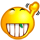,
Trong bài này, chúng ta sẽ tạo ra một sóng hình Sine và lưu nó theo định dạng WAV.
Code mình đã quăng lên một cục từ bài trước, hôm nay chúng ta sẽ bóc tách nó ra để coi cách tạo ra một sóng hình Sine bằng Python và lưu nó dưới định dạng WAV.
Chúng ta bắt đầu nào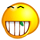:

Đầu tiên chúng ta cần phải import các thư viện cần thiết:
import numpy as np
import wave
import struct
import matplotlib.pyplot as plt

Trong đó:
• numpy là package giúp cho việc tính toán các phép tính khoa học trên Python được dễ dàng hơn.
• wave là module cung cấp interface tương tác với WAVE sound format (định dạng âm thanh WAV).
• struct là module để convert giữa python value và C struct.
• matplotlib.pyplot dùng để vẽ đồ thị sóng Sine được tạo ra.

B1: Tạo sóng hình sin với tần số 1000Hz.
Ta định nghĩa nghĩa trước các thông số của sóng sin được tạo.
sampling_rate = 44100
frequency = 1000
num_samples = 88200
sine_wave = []
Trong đó:
• sampling_rate là tốc độ lấy mẫu = 2 * 22050 = 2 * tần số nghe đươc.
• frequency là tần số sóng Sine.
• num_samples là số lượng mẫu sẽ lấy.
• sine_wave là list lưu các mẫu.
for x in range(num_samples):
sine_wave.append(np.sin(2 * np.pi * frequency * x * 1/sampling_rate))
Với giá trị x chạy từ 0 đến num_samples - 1= 88200 - 1, ta thêm các giá trị được lấy mẫu sin(2*pi*f*n*T) vào list sine_wave.
Trong đó, T là khoảng thời gian liên tiếp giữa hai lần lấy mẫu = 1/sampling_rate, n là số thứ tự của mẫu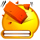.
Để có thể hiểu hơn thì hãy quan sát và ngẫm nghĩ hình sau:Lưu ý: Ta có thể mô tả lệnh trên bằng cách ngắn gọn hơn như sau:
sine_wave = [np.sin(2 * np.pi * frequency * x/sampling_rate) for x in range(num_samples)]
B2: Vẽ kiểm chứng coi cái thứ mình tạo ra đã phải là sóng Sine chưa.
# Plot sine wave
plt.plot(sine_wave[:300])

plt.show()• Nếu bạn để ý thì có thể thấy rằng về hình dạng thì đúng là sóng Sine thật nhưng về tần số thì có gì đó sai sai.
• Theo hình thì chu kì sóng Sine cỡ 44, lấy đâu ra cái tần số 1000Hz.
• Xin hãy chú ý rằng 2 đơn vị liên tiếp trên trục hoành các nhau một khoảng là T = 1/44100(s). Do đó chu kì sóng Sine là 44*1/44100(s) => Tần số sóng Sine cỡ 1/(44*1/44100) = 1002Hz. Vậy nó mới đúng nì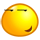.
B3: Lưu sóng Sine với định dạng WAV và tận hưởng bản nhạc do mình code ra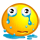.
Đầu tiên, ta phải config một số thông số cho file wav của chúng ta. Các thông số đó bao gồm:​
• nchannels : Số kênh của âm thanh --> 1 - mono audio
• sampwidth : Chiều dài mẫu đơn vị là bytes --> 2
• framerate : Tốc độ lấy mẫu --> sampling_rate = 44100.
• nframes : Số lượng mẫu --> num_samples = 88200
• comptype : compression type, dữ liệu của ta không được nén --> "NONE"
• compname: compression name, dữ liệu của ta không được nén --> "not compressed"
wav_file.setparams((nchannels, sampwidth, int(sampling_rate), nframes, comptype, compname))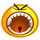??? Phần params này được lưu ở đâu ???Xem lại bài 1 để có câu trả lời.
Sau đó, ta sẽ viết các giá trị lấy mẫu vào file wave:
• Mở file:
file = "test.wav"
wav_file=wave.open(file, 'w')
• Viết vào file:
for s in sine_wave:
wav_file.writeframes(struct.pack('h', int(s*amplitude)))
• Đóng file:
wav_file.close()
Lưu ý 1: struct.pack(format, v1, v2, ...) dùng sẽ trả về một byte object (sequence of Bytes) dựa vào format và các value v1, v2,... Nó giống như hàm int sprintf ( char * str, const char * format, ... ) trong C/C++ với h = hex. Chúng ta cần dùng hàm này để convert Python value về một chuỗi các byte.
Lưu ý 2: Vì sample hiện giờ là số float64 trong đoạn từ -1 đến 1 nên ta cần nhân với amplitude và ép thành kiểu số int.
Lưu ý 3: amplitude phải là một số nằm trong đoạn từ 0 đến 2^15-1 = 32767 vì:
sampwidth = 2 bytes = 16 bits
sample là số có dấu
=> -32768 < sample < 32767.
Bài tập không ai làm:
• Đọc hiểu lại phần 4.b bài 1 để biết cách thêm thêm sóng Sine vào file âm thanh nhằm tạo noise.
• Tạo ra stereo audio, một kênh là sóng Sine 500Hz, một kênh là sóng Sine 10000Hz, biên độ sóng 20000. Đeo tai nghe và thường thức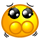. Để xem tai bạn có phân biệt được bên nào tần số cao, bên nào tần số thấp không.
Hết bài 2.

Last edited: May 17, 2019
Chu_Hai_Nam likes this.